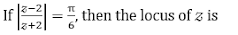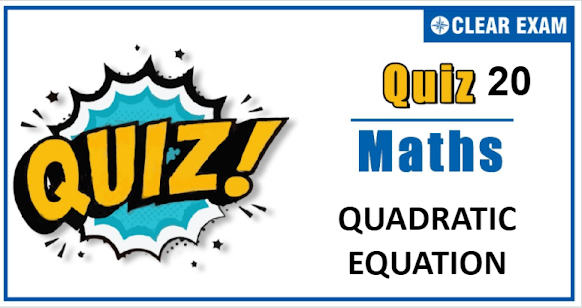## [LATEST]\$type=sticky\$show=home\$rm=0\$va=0\$count=4\$va=0

As per analysis for previous years, it has been observed that students preparing for JEE MAINS find Mathematics out of all the sections to be complex to handle and the majority of them are not able to comprehend the reason behind it. This problem arises especially because these aspirants appearing for the examination are more inclined to have a keen interest in Mathematics due to their ENGINEERING background.

Furthermore, sections such as Mathematics are dominantly based on theories, laws, numerical in comparison to a section of Engineering which is more of fact-based, Physics, and includes substantial explanations. By using the table given below, you easily and directly access to the topics and respective links of MCQs. Moreover, to make learning smooth and efficient, all the questions come with their supportive solutions to make utilization of time even more productive. Students will be covered for all their studies as the topics are available from basics to even the most advanced.

Q1.  The curve represented by Im(z2)=k, where k is a non-zero real number, is
•  A pair of straight lines
•  An ellipse
•  A parabola
•  A hyperbola
Q2.•  A straight line
•  A Circle
•  A parabola
•  An ellipse
Q3.The real root of the equation x3-6x+9=0 is
•  -6
•  -9
•   6
•  -3
Q8. If α,β are the roots of x2+bx-c=0, then the equation whose roots are b and c is
• x2+ax-β=0
• x2-x(α+β+αβ)-α β(α+β)=0
•  x2+(α+β-αβ)x-αβ(α+β)=0
•  x2+(α+β+αβ)x+αβ(α+β)=0
Q9. The number of values of a for which (a2-3a+2)x2+(a2-5a+6)x+a2-4=0 is an identity in x, is
•  0
•  1
•  2
•  3
Q10. Given that ‘a’ is a fixed complex number, and λ′ is a scalar variable, the point z satisfying z=a(1+iλ) traces out
•  A straight line through the point ′a′
•  A circle with centre at the point ′a′
•  A straight line through the point ′a′ and perpendicular to the join 0 and that point ′a′
•  None of these## Want to know more

Please fill in the details below:

## Latest NEET Articles\$type=three\$c=3\$author=hide\$comment=hide\$rm=hide\$date=hide\$snippet=hide

Name

ltr
item
BEST NEET COACHING CENTER | BEST IIT JEE COACHING INSTITUTE | BEST NEET & IIT JEE COACHING: Quadratic_equation_Quiz-20